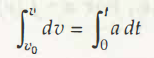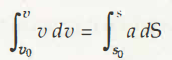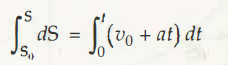To find out the final equation for constant acceleration, the following formulations are:

S = S0

t =0

v =v0At point (1.2), the equation stands at dv = a dt

On integrating this equation, the calculation is,

An alternative to this calculation is v – v0 = at

v =v0 +atAgain on integrating on the seconds instance, the calculation stands to be,

The next step in this is,

v2 =v02 +2a (S –S0)

From the equation (1.1),

dS =v  dt

dS = (v0+at) dtso integration equation as per the above is,

S –S0 = v0 t +1/2 at2

S =S0 + v0 t +1/2 at2

P.S.

It is only in case of constant acceleration when equations (1.5), (1.6) and (1.7) are required to be applied.

Links of Next Mechanical Engineering Topics:-### Customer Reviews

My Homework Help
Rated 5.0 out of 5 based on 510 customer reviews at